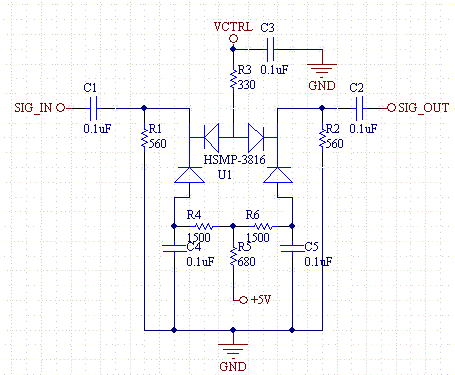# Variable PIN Diode Attenuator

This is a very useful RF attenuator circuit which works from 300KHz to the 3GHz range based on the quad PIN diode array HSMP-3816 from Avego. The lower PIN diodes are biased at 1mA which gives about a 40 to 70 ohm impedance. R5 can be increased to increase the RF input and output impedances of the circuit.

The upper diodes are biased by the control voltage which varies between 0 and 10V. The signal is essentially blocked when the control voltage is 0V and passes when the control voltage is high. The beauty of this circuit is that a small current can control a higher power RF waveform.

To create a control voltage of 0 to 10V use a voltage servo circuit.Schematic of Variable RF Attenuator based on a Quad PIN Diode